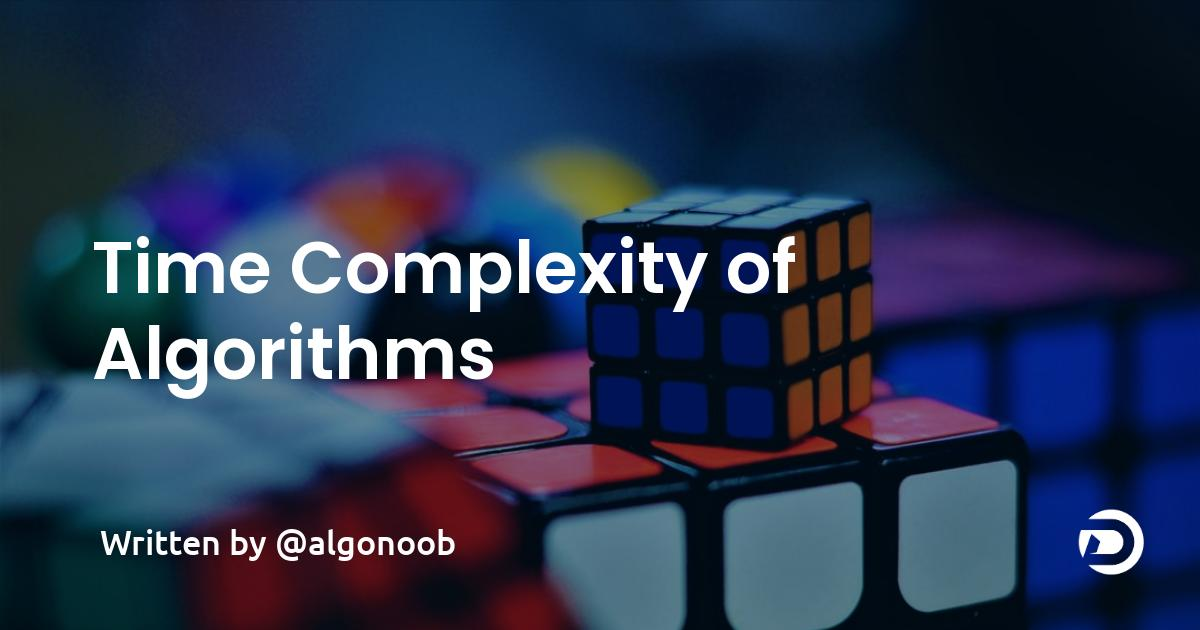# Time Complexity of Algorithms

Written by Richa Kiran on Aug 19th, 2021 Views

The time complexity of an algorithm is the time taken by an algorithm to run.

## The significance

Sometimes, when we try to solve a problem, we might think of more than one way to solve it. Now some of these approaches might take less time to come to the solution while the others might take more time.

The approach that takes the least time is the best approach possible for that particular problem. Whereas, the approach taking the most time is the worst solution for it. All the other approaches will take time ranging between the time taken by the best and the worst cases.

## An example

Let's take an example to understand it better. Suppose that you're given an array of random numbers. You're supposed to arrange them in the increasing order.

How would you go about it?

Let's say, you try to do it this way -

1. Set x as 0 (the index of the first unsorted element of the array).
2. Scan the array from indices x to end of the array.
3. Find the smallest element, and swap it with the element at x.
4. Increase x by 1
5. Repeat the above steps again and again until no element is left or x = last index.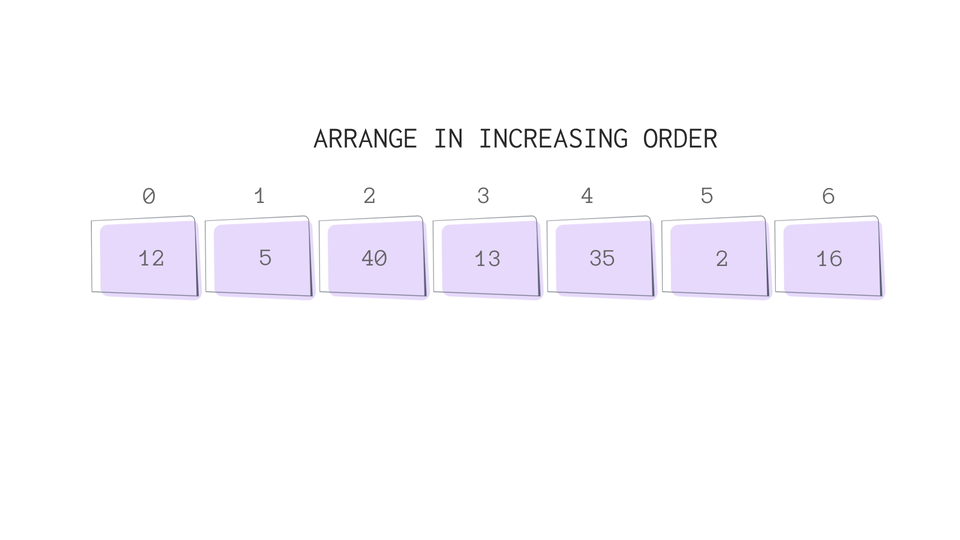Can you think of a scenario in which the above algorithm would take the most time? or a scenario wherein it would take the least time?

Let's say that each scan takes n unit of time(n being the number of elements we're trying to scan) and swap operation requires 1 unit of time. The following is the best case scenario for this approach-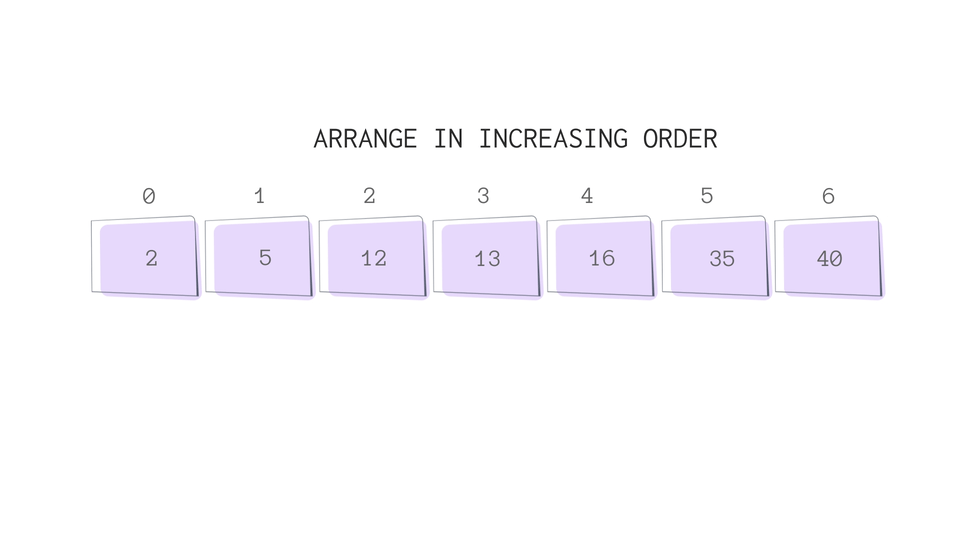As you can see the time complexity comes out to be 27 units in this case.

Coming to the worst case. That would happen if the array was oppositely arranged(in this case in the decreasing order) initially.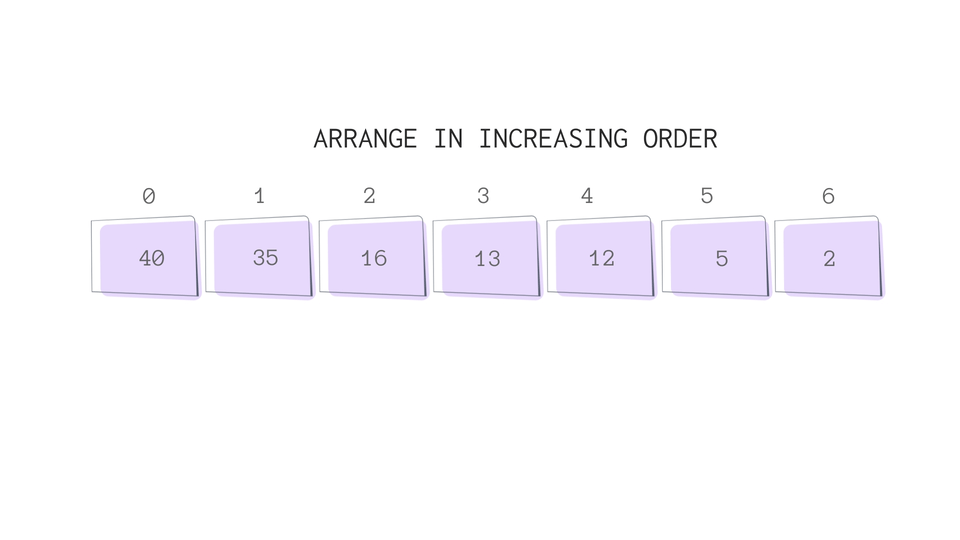Here, the time comes out to be 30 units.

Through this I just want to make you understand the literal meaning of time complexity of an algorithm. The above is not an accurate way to calculate the time complexity. It was just for the sake of your understanding.

Our main aim in this whole process is to find out a way to solve this problem in such a way that the time complexity or the time taken by an algorithm in the worst case possible is the least as compared to other available methods.

## Why focus on the worst case?

The reason for considering the worst case scenario is that the maximum time an algorithm would take would be in the worst case. If we make the worst case take as less time as possible, the algorithm would be considered more efficient.

Let's say that there's a group of people running in a race. We're given the task of calculating the average speed of the group. Now, the average speed of the group would decrease if the pace of the slowest runner decreases(provided that the speed of other runners is uniformly distributed in the range of speeds). Consider another group running alongside this group, but its slowest runner is running faster than the first group. Obviously, the second group would be considered a winner in terms of the average speed.

If you apply the same analogy to the time complexities of an algorithm, the lower the time complexity of the worst case, the better the overall algorithm.

## Plotting it on the Graph

If you try to plot all these complexities on a graph. You'll see that the worst case forms the "Upper bound" or the "Upper limit" of the time complexities and the best case forms the "Lower bound" or the "Lower limit". A typical graph of time complexities of an algorithm looks like this -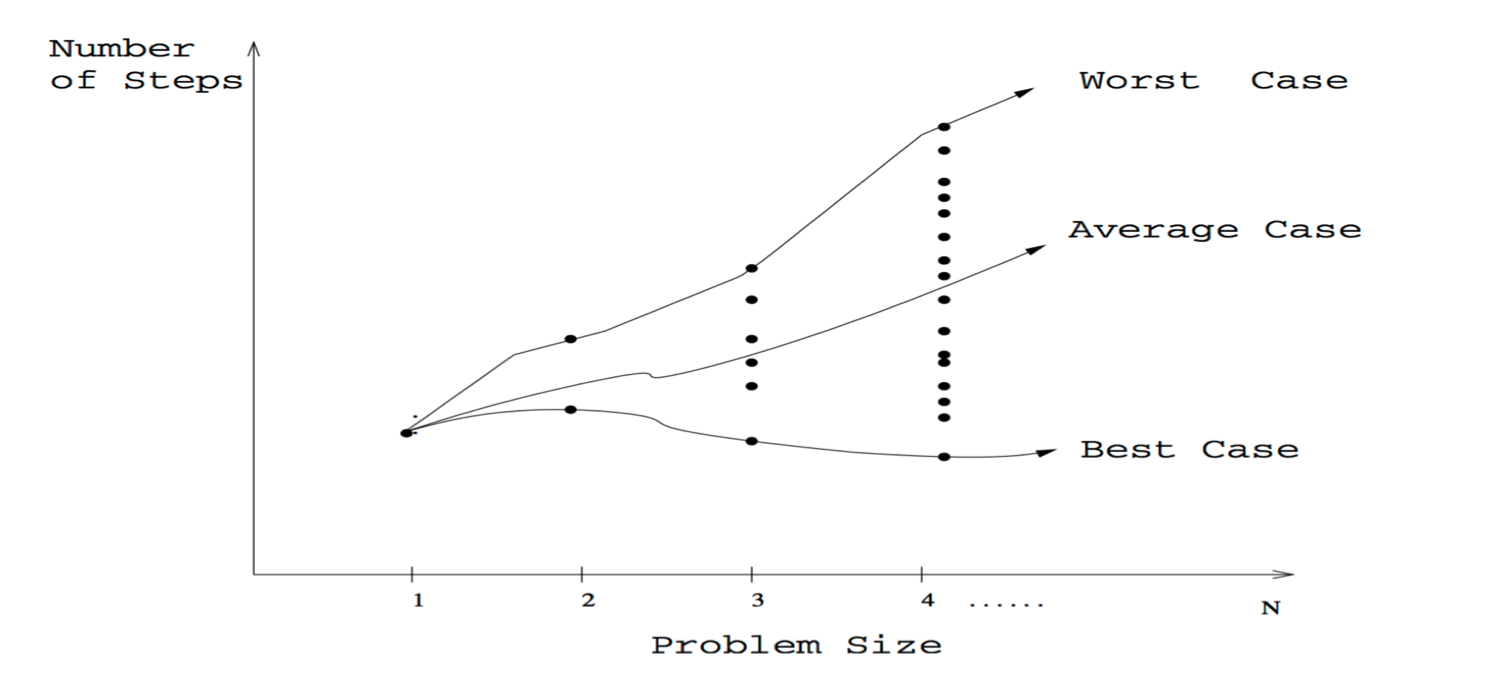## The end.

I hope you got some basic idea of the concept of time complexity through this article. You can check out some other articles on data structures here.